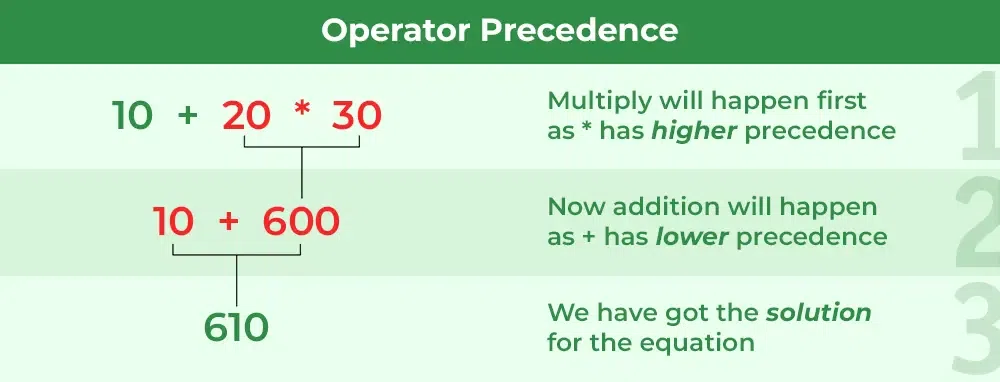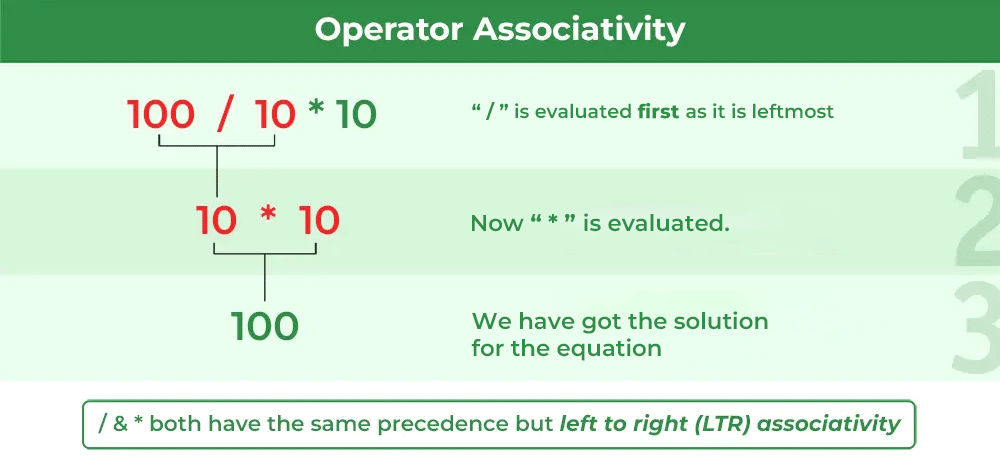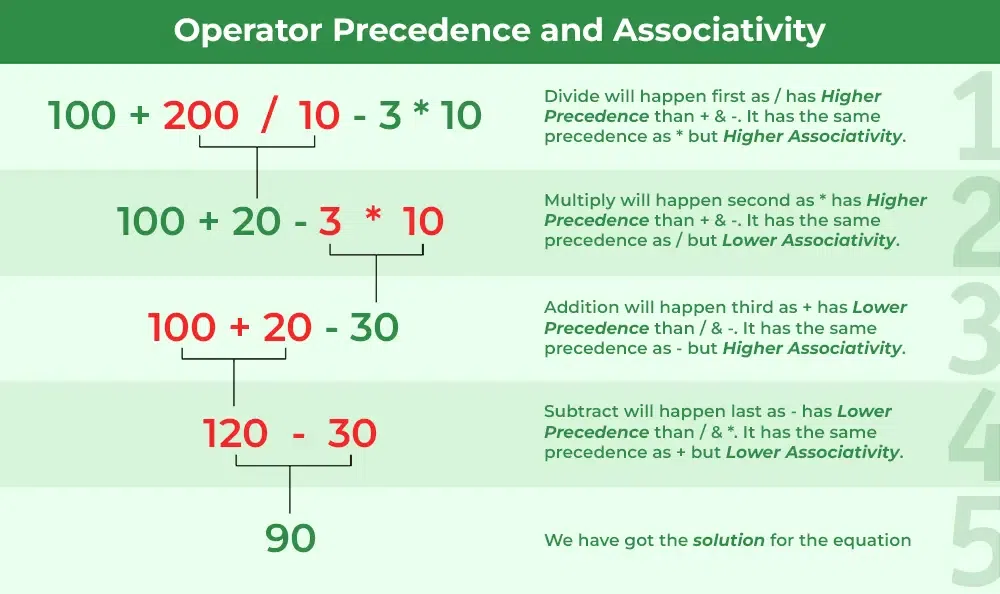Open In App
Related Articles
• Write an Interview Experience
• C Programming Language Tutorial

# Operator Precedence and Associativity in C

The concept of operator precedence and associativity in C helps in determining which operators will be given priority when there are multiple operators in the expression. It is very common to have multiple operators in C language and the compiler first evaluates the operater with higher precedence. It helps to maintain the ambiguity of the expression and helps us in avoiding unnecessary use of parenthesis.

In this article, we will discuss operator precedence, operator associativity, and precedence table according to which the priority of the operators in expression is decided in C language.

## Operator Precedence and Associativity Table

The following tables list the C operator precedence from highest to lowest and the associativity for each of the operators:

## Operator Precedence in C

Operator precedence determines which operation is performed first in an expression with more than one operator with different precedence.

### Example of Operator Precedence

Let’s try to evaluate the following expression,

`10 + 20 * 30`

The expression contains two operators, + (plus), and * (multiply). According to the given table, the * has higher precedence than + so, the first evaluation will be

`10 + (20 * 30)`

After evaluating the higher precedence operator, the expression is

`10 + 600`

Now, the + operator will be evaluated.

`610`We can verify this using the following C program

## C

 `// C Program to illustrate operator precedence``#include ` `int` `main()``{``    ``// printing the value of same expression``    ``printf``(``"10 + 20 * 30 = %d"``, 10 + 20 * 30);` `    ``return` `0;``}`

Output

`10 + 20 * 30 = 610`

As we can see, the expression is evaluated as,10 + (20 * 30) but not as (10 + 20) * 30 due to * operator having higher precedence.

## Operator Associativity

Operator associativity is used when two operators of the same precedence appear in an expression. Associativity can be either from Left to Right or Right to Left.

### Example of Operator Associativity

Let’s evaluate the following expression,

`100 / 5 % 2`

Both / (division) and % (Modulus) operators have the same precedence, so the order of evaluation will be decided by associativity.

According to the given table, the associativity of the multiplicative operators is from Left to Right. So,

`(100 / 5) % 2`

After evaluation, the expression will be

`20 % 2`

Now, the % operator will be evaluated.

`0`We can verify the above using the following C program:

## C

 `// C Program to illustrate operator Associativity``#include ` `int` `main()``{``    ``// Verifying the result of the same expression``    ``printf``(``"100 / 5 % 2 = %d"``, 100 / 5 % 2);` `    ``return` `0;``}`

Output

`100 / 5 % 2 = 0`

Operators Precedence and Associativity are two characteristics of operators that determine the evaluation order of sub-expressions.

## Example of Operator Precedence and Associativity

In general, the concept of precedence and associativity is applied together in expressions. So let’s consider an expression where we have operators with various precedence and associativity

`exp = 100 + 200 / 10 - 3 * 10`

Here, we have four operators, in which the / and * operators have the same precedence but have higher precedence than the + and operators. So, according to the Left-to-Right associativity of / and *, / will be evaluated first.

```exp = 100 + (200 / 10) - 3 * 10
= 100 + 20 - 3 * 10```

After that, * will be evaluated,

```exp = 100 + 20 - (3 * 10)
= 100 + 20 - 30```

Now, between + and , + will be evaluated due to Left-to-Right associativity.

```exp = (100 + 20) - 30
= 120 - 30```

At last, will be evaluated.

```exp = 120 - 30
= 90```Again, we can verify this using the following C program.

## C

 `// C Program to illustrate the precedence and associativity``// of the operators in an expression``#include `  `int` `main()``{``    ``// getting the result of the same expression as the``    ``// example``    ``int` `exp` `= 100 + 200 / 10 - 3 * 10;``    ``printf``(``"100 + 200 / 10 - 3 * 10 = %d"``, ``exp``);`  `    ``return` `0;``}`

Output:

100 + 200 / 10 – 3 * 10 = 90

## Important Points

There are a few important points and cases that we need to remember for operator associativity and precedence which are as follows:

### 1. Associativity is only used when there are two or more operators of the same precedence.

The point to note is associativity doesn’t define the order in which operands of a single operator are evaluated. For example, consider the following program, associativity of the + operator is left to right, but it doesn’t mean f1() is always called before f2(). The output of the following program is in-fact compiler-dependent.

Example

## C

 `// Associativity is not used in the below program.``// Output is compiler dependent.` `#include ` `int` `x = 0;``int` `f1()``{``    ``x = 5;``    ``return` `x;``}``int` `f2()``{``    ``x = 10;``    ``return` `x;``}``int` `main()``{``    ``int` `p = f1() + f2();``    ``printf``(``"%d "``, x);``    ``return` `0;``}`

Output

`10 `

See this for details.

### 2. We can use parenthesis to change the order of evaluation

Parenthesis ( ) got the highest priority among all the C operators. So, if we want to change the order of evaluation in an expression, we can enclose that particular operator in ( ) parenthesis along with its operands.

Example

Consider the given expression

```100 + 200 / 10 - 3 * 10
= 90```

But if we enclose 100 + 200 in parenthesis, then the result will be different.

```(100 + 200) / 10 - 3 * 10 = 0
= 0```

As the + operator will be evaluated before / operator.

### 2. All operators with the same precedence have the same associativity.

This is necessary, otherwise, there won’t be any way for the compiler to decide the evaluation order of expressions that have two operators of the same precedence and different associativity. For example + and – have the same associativity.

### 3. Precedence and associativity of postfix ++ and prefix ++ are different.

The precedence of postfix ++ is more than prefix ++, their associativity is also different. The associativity of postfix ++ is left to right and the associativity of prefix ++ is right to left. See this for examples.

### 4. Comma has the least precedence among all operators and should be used carefully.

For example, consider the following program, the output is 1.

Example

## C

 `#include ``int` `main()``{``    ``int` `a;``    ``a = 1, 2, 3; ``// Evaluated as (a = 1), 2, 3``    ``printf``(``"%d"``, a);``    ``return` `0;``}`

Output

`1`

See this and this for more details.

### 5. There is no chaining of comparison operators in C

In Python, an expression like “c > b > a” is treated as “c > b and b > a”, but this type of chaining doesn’t happen in C. For example, consider the following program. The output of the following program is “FALSE”.

Example

## C

 `#include ``int` `main()``{``    ``int` `a = 10, b = 20, c = 30;` `    ``// (c > b > a) is treated as ((c  > b) > a), associativity of '>'``    ``// is left to right. Therefore the value becomes ((30 > 20) > 10)``    ``// which becomes (1 > 10)``    ``if` `(c > b > a)``        ``printf``(``"TRUE"``);``    ``else``        ``printf``(``"FALSE"``);``    ``return` `0;``}`

Output

`FALSE`

## Conclusion

It is necessary to know the precedence and associativity for the efficient usage of operators. It allows us to write clean expressions by avoiding the use of unnecessary parenthesis. Also, it is the same for all the C compilers so it also allows us to understand the expressions in the code written by other programmers.

Also, when confused about or want to change the order of evaluation, we can always rely on parenthesis ( ). The advantage of brackets is that the reader doesn’t have to see the table to find out the order.